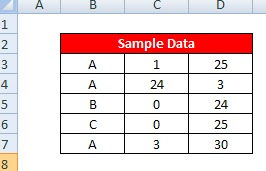# Return Multiple Values For Lookup Value

This tutorial explains how to get multiple values for a single lookup value.

Sample DataMultiple Values with Vlookup

OutputOutput Snapshot

Formula

Enter the following formula in G9 and paste it down and to the right
=INDEX(C\$3:C\$7, SMALL(INDEX((\$F\$9=\$B\$3:\$B\$7)*(MATCH(ROW(\$B\$3:\$B\$7), ROW(\$B\$3:\$B\$7)))+(\$F\$9<>\$B\$3:\$B\$7)*1048577, 0, 0), ROW(A1)))
C\$3:C\$7 - Desired column you want the formula to return
B\$3:B\$7 - Lookup column
F9 - Lookup value1.